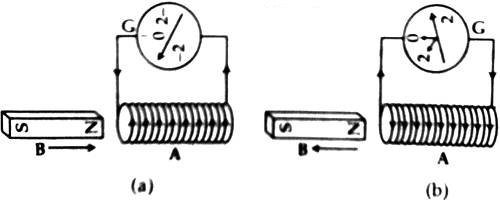# Production of Electricity by a Magnet

Production of Electricity by a Magnet

The mechanical energy moves the wire into the magnetic field of the magnet so that the wire cuts through the magnetic lines of force. As a result, electric current is produced. We know that that electric energy is produced due to the relative motion between a magnet and a coil. Scientist Faraday first presented the production of induced current by an experiment shown below.

Suppose NS is a bar magnet, A is a closed coil in which a galvanometer is connected [Figure]. The presence of current can be seen from the deflection of the galvanometer needle. The coil is made by thin insulated wire. Now, if the north pole of the magnet is taken slowly towards the edge of the coil, it will be seen that the galvanometer needle is deflected [Fig. (a)]. So, the existence of current in the coil is proved. If the same pole is moved away from the coil the galvanometer needle will give deflection in the opposite direction [Fig. (b)].If the magnet is stopped the galvanometer will come to stationary state 0. So, it is proved that as long as there is a relative motion between the magnet and the coil, there is an induced current and electric energy is produced.